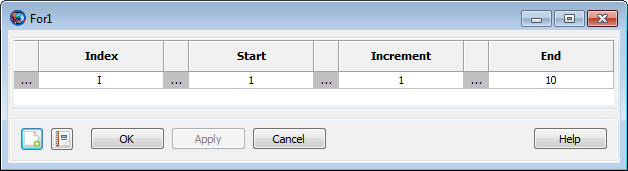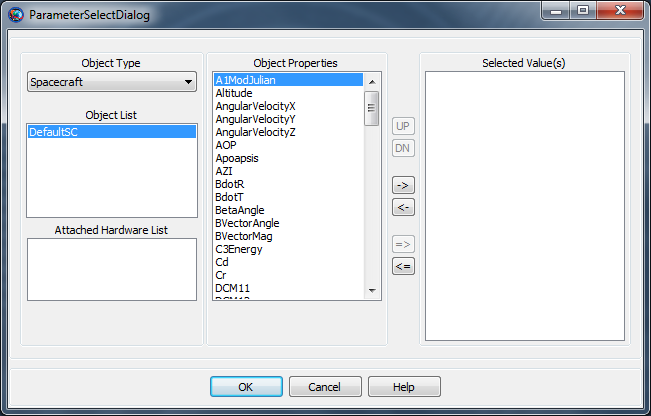# For

## For

For — Execute a series of commands a specified number of times

## Script Syntax

````For` `Index` = `Start`:[`Increment`:]`End`
[`script statement`]
…
`EndFor````

## Description

The For command is a control logic statement that executes a series of commands a specified number of times. The command argument must have one of the following forms:

`Index` = `Start`:`End`

This syntex increments Index from Start to End in steps of 1, repeating the script statements until Index is greater than End. If Start is greater than End, then the script statements do not execute.

`Index` = `Start`:`Increment`:`End`

This syntax increments Index from Start to End in steps of Increment, repeating the script statements until Index is greater than End if Increment is positive and less than End if Increment is negative. If Start is less than End and Increment is negative, or if Start is greater than End and Increment is positive, then the script statements do not execute.

See Also: If, While

## Options

Option Description
Index

Independent variable in a for loop. Index is computed according to the arithmetic progression defined by the values for Start, Increment, and End.

 Accepted Data Types Variable Allowed Values -∞ < Index < ∞ Default Value Variable named I Required yes Interfaces GUI, script
Start

Initial value for the Index parameter

 Accepted Data Types parameter Allowed Values -∞ < Start < ∞ Default Value 1 Required yes Interfaces GUI, script
Increment

The Increment parameter is used to compute the arithmetic progression of the loop Index such that pass i through the loop is Start + i* Increment if the resulting value satisfies the constraint defined by End.

 Accepted Data Types parameter Allowed Values -∞ < Increment < ∞ Default Value 1 Required no Interfaces GUI
End

The End parameter is the upper (or lower if Increment is negative) bound for the Index.

 Accepted Data Types parameter Allowed Values -∞ < End < ∞ Default Value 10 Required yes Interfaces GUI, script

## GUIThe For command GUI panel contains fields for all of its parameters: Index, Start, Increment, and End. To edit the values, click the field value you wish to change and type the new value (e.g. `5`, `anArray(1,5)`, or `Spacecraft.X`). Alternately, you can either right-click the field value or click the ellipses () button to the left of the field. This displays the ParameterSelectDialog window, which allows you to choose a parameter from a list.## Remarks

The values of the Index, Start, Increment, and End parameters can be any of the following types:

• Literal numeric value (e.g. 1, 15.2, -6)

• Variable resource

• Array resource element

• Resource parameter of numeric type (e.g. Spacecraft.X, ChemicalThruster.K1)

with the extra requirement that if a Resource parameter is used for Index, the parameter must be settable.

The index specification cannot contain mathematical operators or parentheses. After execution of the For loop, the value of Index retains its value from the last loop iteration. If the loop does not execute, the value of Index remains equal to its value before the loop was encountered.

Changes made to the index variable inside of a For loop are overwritten by the For loop statement. For example, the output from the following snippet:

```        ```For I = 1:1:3
I = 100
Report aReport I
EndFor```
```

is:

```100
100
100 ```

Changes made to the the Start, Increment, and End parameters made inside of a loop do not affect the behavior of the loop. For example, the output from the following snippet:

```        ```J = 2
K = 2
L = 8
For I = J:K:L
J = 1
K = 5
L = 100
Report aReport I
EndFor```
```

is:

```2
4
6
8   ```

## Examples

Propagate a spacecraft to apogee 3 times:

```          ```Create Spacecraft aSat
Create Propagator aPropagator
Create Variable I

BeginMissionSequence

For I = 1:1:3
Propagate aPropagator(aSat, {aSat.Apoapsis})
EndFor```
```

Index into an array:

```          ```Create Variable I J
Create Array anArray[10,5]
BeginMissionSequence

For I = 1:10
For J = 1:5
anArray(I,J) = I*J
EndFor
EndFor```
```